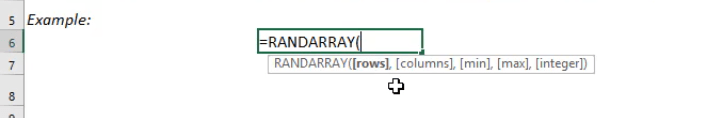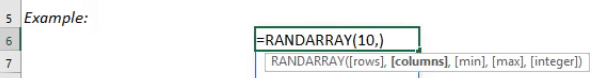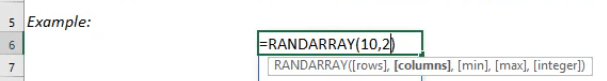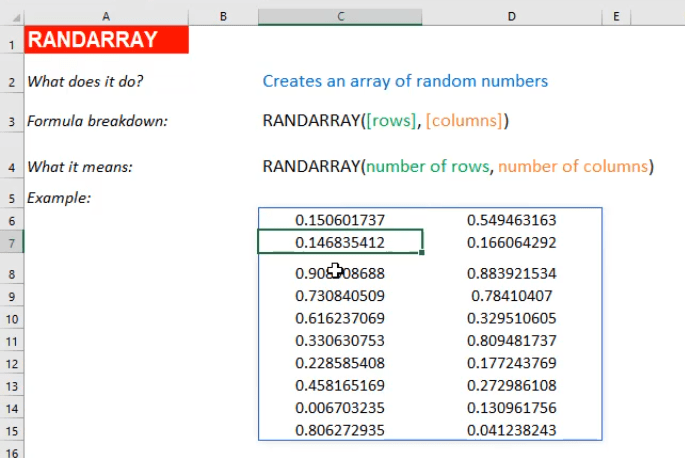What does it do?

Creates an array of random numbers

Formula breakdown:

=RANDARRAY([rows], [columns])

What it means:

=RANDARRAY(number of rows, number of columns)

Did you know that you can now generate random numbers in an array? Yes you can! It is definitely possible now with Excel’s RANDARRAY Formula. It is a new formula introduced in Office 365 released in 2018!

It returns random values between 0 and 1 by default. I explain how you can do this below:STEP 1: We need to enter the RANDARRAY function in a blank cell:

## =RANDARRAY(STEP 2: The RANDARRAY arguments:

## [rows]

How many rows to fill random values with?

Let us go for 10 rows.

## =RANDARRAY(10,## [columns]

How many columns to fill random values with?

Let us go for 2 columns.

## =RANDARRAY(10, 2)Now we have our 10 x 2 area filled with random values between 0 and 1!How to Use the RANDARRAY Formula in Excel